序号 预设故障区段 FTU上传故障信息 定位过程 定位结果 1 区段(8) [1 1 0 0 1 0 1 −1 1 −1] ${p}_{99}=1$ ， ${p}_{9,10}=1$ ， ${p}_{10,10}=-1$ 区段(8) 2 区段(6) [1 1 0 0 1 1 −1 −1 −1 −1] ${p}_{66}=1$ 区段(6) 3 区段(5) [1 1 0 0 1 0 −1 −1 −1 −1] ${p}_{55}=1$ ， ${p}_{56}={p}_{57}=1$ ， 区段(5) ${p}_{66}\ne {p}_{77}$ ， ${p}_{66}={p}_{77}=-1$ ， ${p}_{55}=1$ ， ${p}_{56}=1$ ， ${p}_{66}=-1$ ${p}_{55}=1$ ，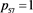，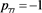4 区段(7) [1 1 0 0 1 0 1 −1 −1 −1]，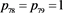， 区段(7)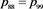，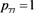，，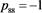，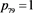，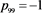5 区段(1) (8) [1 −1 0 0 −1 0 −1 −1 1 −1]，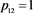，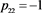区段(1)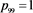，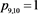，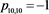区段(8)